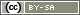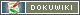# valueList()

`array valueList(string question ID, [array items], [string output format])`
`array valueList(array variables, [null], [string output format])`
`array valueList(string system ID)`

The function valueList() retrieves the answer codes for all items of a question – or for all items of a question if no items are specified –. Alternatively, a list of variables can be specified, for which the answer codes are retrieved.

• Question ID
The ID of a question in the question catalogue.
• Items
Optionally, a list of items can be specified. If no items are specified, the question uses all items of the question.
• Variables
Instead of a question ID, a list of variables can also be specified,
e.g. `valueList(array('AB01', 'AB02_01', 'AB02_02'))`'.
• System ID
The ID `'TIME`' retrieves the dwell time of the pages (TIME001, TIME002, etc.) as an array with page numbers as index and dwell time [seconds] as value.
• output format
The output format determines whether the function returns the response code (default) or the caption text of the response. The same specifications are possible as for `value()`.

## Return Value

The function `valueList()` returns an associative array. The variable IDs serve as keys, the response codes for the items as values, e.g.

```array(
'AB01' => 3,
'AB02_01' => -1,
'AB02_02' => 2
)```

## Random Generator

A frequent use of the function `valueList()` is in connection with a Zufallsgenerator, which draws several ballots per interview.

The following PHP code determines the codes of all ballots drawn in the random generator “IV01” as Array.

`\$codes = valueList('IV01');`

The following code reads the labels of the drawn ballots instead of their numeric codes:

`\$codes = valueList('IV01', null, 'label');`

## Items with the highest rating

Another example of the use of valueList() is the selection of items … not with a fixed criterion like `getItems()`, but e.g. the three items with the highest scores.

Let's take as an example a battery of sliders (question of type slider, e.g. “SR01”) with 15 items. In a follow-up question, only those three items that have received the highest evaluation from the participant (e.g., usage quantity) shall be examined in greater detail.

With `valueList()` first a list of all answers is retrieved. Then this list is sorted in descending order – using `arsort()`, so that the assignment of variable ID and value is preserved.

```\$values = valueList('SR01');
arsort(\$values);```

The variable `\$values` could now contain e.g. the following

KeyValue
SR01_07 101
SR01_02 63
SR01_04 59
SR01_15 59
SR01_10 42
SR01_11 38
SR01_05 35
SR01_14 32

Caution: It can happen now that the third and fourth item (in the example above “SR01_04” and “SR01_15”) got the same value. You must decide how to deal with this case: Should the fourth item appear in the follow-up question? Should only one of the items be used by coincidence? For this example, exactly three items should always be queried, so one of the two items must be selected at random. This is not quite trivial. Therefore the list is divided into three parts: Items that reach a higher value than the third item (`SR01_04 ⇒ 59`, `\$threshold`) (`\$greater`), items that reach exactly this value (`\$equal`) and items that reach a lower value. We don't need the last list anymore.

```\$order = array_keys(\$values);
\$threshold = \$values[\$order];
\$greater = array();
\$equal = array();
foreach (\$values as \$varID => \$value) {
\$itemID = (int)ltrim(substr(\$varID, 5), "0");
if (\$value > \$threshold) {
\$greater[] = \$itemID;
} elseif (\$value == \$threshold) {
\$equal[] = \$itemID;
}
}```

To explain `\$threshold`: The variable `\$order` contains the keys of the array `\$values`. This allows to retrieve the key of the third value (index 2) in the sorted list. So `\$order` would be “SR01_04” and if you retrieve this key from `\$values`, you get the `59` in the example.

To explain vin `\$itemID`: We do not need the complete variable ID (e.g. “SR01_01”), but only the number of the item, e.g. `1`. Therefore we first take everything from the sixth position (index 5), i.e. “01”, using `substr()` from “SR01_01”, and then we remove the leading “0” using `ltrim()`, so that PHP can convert the text “1” into the number `1` using `(int)`.

The shuffle of the list `\$equal` is done with `shuffle()`. And from this list we need three minus the number of elements from the list `\$greater`, determined via `count()`.

```shuffle(\$equal);
\$needed = 3 - count(\$greater);
\$items = array_merge(\$greater, array_slice(\$equal, 0, \$needed));```

The \$items list now contains the numbers of the top 3 items, either `7,2,4` or `7,2,15`. These can now be queried in a follow-up question which contains the same 15 items as the question “SR01” using `question()`.

`question('FF02', \$items);`

The complete PHP code will look like this.

```\$values = valueList('SR01');
arsort(\$values);

\$order = array_keys(\$values);
\$threshold = \$values[\$order];
\$greater = array();
\$equal = array();
foreach (\$values as \$varID => \$value) {
\$itemID = (int)ltrim(substr(\$varID, 5), "0");
if (\$value > \$threshold) {
\$greater[] = \$itemID;
} elseif (\$value == \$threshold) {
\$equal[] = \$itemID;
}
}

shuffle(\$equal);
\$needed = 3 - count(\$greater);
\$items = array_merge(\$greater, array_slice(\$equal, 0, \$needed));

question('FF02', \$items);```